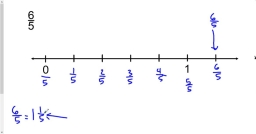Find whole

Find whole numbers between 155 and 232 is divisible by 2, 5 and 10.

x1 =  160
x2 =  170
x3 =  180
x4 =  190
x5 =  200
x6 =  210
x7 =  220
x8 =  230

Step-by-step explanation:Did you find an error or inaccuracy? Feel free to write us. Thank you!Tips to related online calculators
Do you want to calculate least common multiple two or more numbers?
Do you want to perform natural numbers division - find the quotient and remainder?